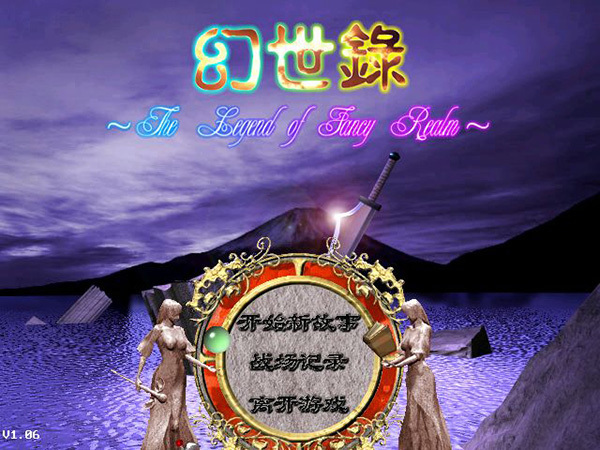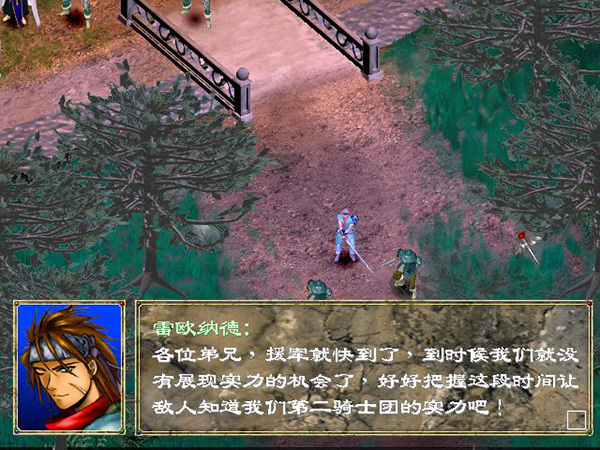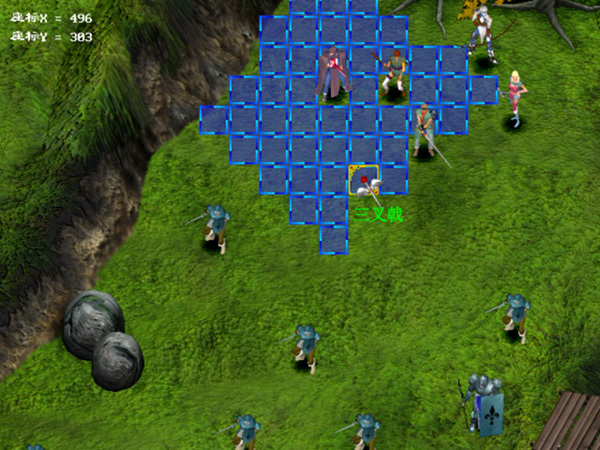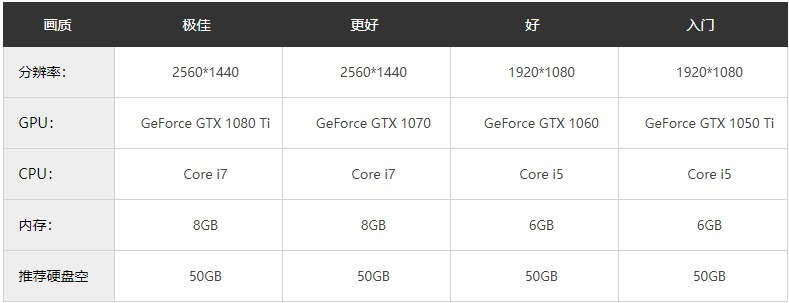# 幻世录1中文版下载-幻世录1硬盘版破解版下载 v1.06

2022年2月13日10:56:54幻世录1中文版下载-幻世录1硬盘版破解版下载 v1.06已关闭评论2阅读模式### 游戏攻略

1、物品：在这里可以随时换装备和对自己或他人使用物品。

2、资料：其实想看某人资料的话直接点移动后再点他就行了，但加点的话只能在这里加。

3、待机：不用解释了吧（当某一人行动结束后，可对它使用“延续之炎”来让他再次行动）

4、移动：即走格，范围多大由移动距离决定，移动距离可以通过转职和装备来增加

5、攻击：使用普攻和各种特殊技攻击，普攻和防御攒气，特殊技耗气（耗气比例就是那个百分之多少）

6、魔法：施放魔法（到了第二三轮你会发现魔法才是通关的王道，不然清兵太慢了）

7、特殊技：技能攻击，需要气力。

1、 -godset：启动密技模式，功能如下

F9：看开头动画

F10：看打倒魔神动画

Alt+Enter：全屏幕/视窗模式切换 (1.01版以上)战场宝能

F5：HP，MP，ST全满

F6：HP为1， MP，ST为0， 可以选择“移动”命令查看敌人状态时使用

F7：第一次经验值等于升级经验值，以后每按一次加100

F8：每按一次加钱10000

2、 -random：读取战场存档后战斗结果会是乱数

3、 -nosfx：关闭音效

4、 -pos：显示游标坐标，方便找宝物

5、 -walker n：设定大地图模式下走路的角色(n=0-8)

1为缇娜、2为琥、3为汉克斯、4为雪拉、5为雷特、6为嚎、7为咕噜、8为克罗蒂

6、 -window：以视窗模式进行游戏，执行速度会比较慢，Win95显示模式需为16bit (1.01版以上)

7、 -page：如果画面会停住(或看不见)才使用 (1.01版以上)### 幻世录1隐藏物品攻略大全

1、 双头的死神(欧姆村)：X = 656, Y = 560 玻璃戒指、回复药、解毒草

2、 遭遇(戈尔山道)：X = 496, Y = 464阔刃剑、回复药 X = 176, Y = 464 妖精之泉x2、

3、 御之源 巢窟(盗贼洞窟)：X = 624, Y = 368 黄金首饰

4、 猛拧(呼啸平原)：X = 304, Y = 592 土之源

5、 宣战(席达镇)：X = 400, Y = 336 魔之源、黄金首饰 X = 816, Y = 688 烈炎戒指、力之源

6、 寂寥(宁静之森)：X = 592, Y = 592 银制发饰、解毒草 X = 784, Y = 368 凝气之环

7、 哀怨(帕尼西雅城 废墟)：X = 816, Y = 240 水晶剑、琥珀水晶

8、 狙兵(菲纳斯河畔)：X = 496, Y = 304 三叉戟 X = 1392, Y = 144 风之源、水之源

9、 失落(废都 曼多力亚)：X = 1296, Y = 560 黄金的圣杯

10、寻(艾瓦台地)：X = 624, Y = 144 湛蓝水晶、土之源 X = 432, Y = 560 深红之瞳

11、援救(艾瓦台地)：X = 624, Y = 144 逆十字 X = 432, Y = 560 火红水晶、灵之源

12、真理(利鲁玛山地)：克里夫未死 X = 48, Y = 80 X = 1488, Y = 208 X = 592, Y = 720 幸运缎带 凝气之环 秘药、妖精之泉

13、真理(利鲁玛山地)：克里夫死亡 X = 48, Y = 80 X = 1488, Y = 208 X = 432, Y = 944 凝气之环 幸运缎带 奥义之证

14、怒涛(巴瀚纳海峡)：X = 1360, Y = 1552 冥晦之轮

15、异种(深渊之沼)：X = 752, Y = 48 ??绿结晶

16、魔骑士(回音之谷)：X = 144, Y = 48 刚岩之盔 X = 144, Y = 432 琥珀水晶

17、狂砂(哈莫特沙漠)：回音之谷打倒克罗蒂 X = 592, Y = 912 女神之冠

18、狂砂(哈莫特沙漠)：回音之谷脱离 X = 112, Y = 656 女神之冠

19、信念(约瑟河)：X = 912, Y = 304 翠绿水晶、水之源 X = 144, Y = 624 极光护轮

20、离散(绝望之谷)：X = 752, Y = 176 火之源、风之源 X = 336, Y = 48 大地之衣

21、奔逃(漆黑之森)：X = 176, Y = 560 邪眼假面 X = 592, Y = 496 湛蓝水晶

22、解明(黄昏之丘 阴)：X = 240, Y = 304 ??黑水晶

23、阻碍(拉格纳沼地)：X = 336, Y = 688 翠绿水晶

24、虚实(沙罗尼亚近郊)：X = 576, Y = 704 丈八蛇矛 X = 640, Y = 160 琥珀水晶

25、变节(萨鲁司海岸)：X = 304, Y = 560 火红水晶 X = 112, Y = 1072 奥义之证

26、未知(古代神殿遗迹)：X = 272, Y = 144 黄金的圣杯

27、崩陷(黄昏之丘 阳)：X = 112, Y = 272 隐密之轮

28、恶魔者之翼(悲叹之湖)：X = 80, Y = 560 圣骑士勋章 X = 1200, Y = 880 支配之杖

29、猎杀(尼布鲁瀑布)：X = 912, Y = 240 恶魔晶石 X = 1168, Y = 976 追风之羽

30、破浪(日没湾)：X = 80, Y = 496 海龙之弓

31、遗产(众神的宫殿遗址)：X = 880, Y = 752 白光之翼 X = 528, Y = 1872 穹苍之炼

32、深邃之间(幽暗坟场)：X = 272, Y = 656 星尘之冠

33、辉煌(龙之息)：X = 48, Y = 816 舞空之靴

34、残光(亚修顿大桥：X = 1104, Y = 1008 旭日之盔### 幻世录1加点攻略

【雷欧纳德】

【缇娜】

【琥】【汉克斯】

【雪拉】

【雷特】

【嚎】

【克罗蒂】

【合体后的咕噜】

### 配置要求